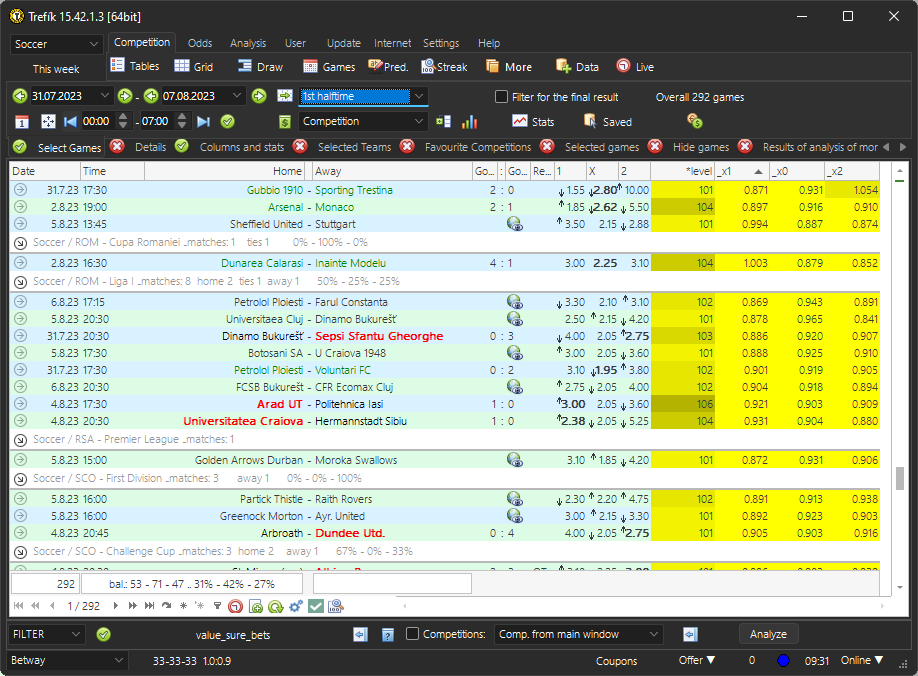# Valuebets in the Offer window

Comparing fresh odds with average odds in Trefík

Trefík offers a search for valuebets. By default, they are calculated in Trefík using the real odds and their difference compared to the average odds from all bookies in Trefík. Value bets can be viewed in Odds / Valuebets window. The same results can be achieved in the Offer window using a filter.

In this case, the valuebet is calculated from the average odds for the given tip for all bookies in Trefík. It is therefore necessary to activate the calculation of average odds in the filter. This can be done by activating the Odds / Mixed Odds Level section: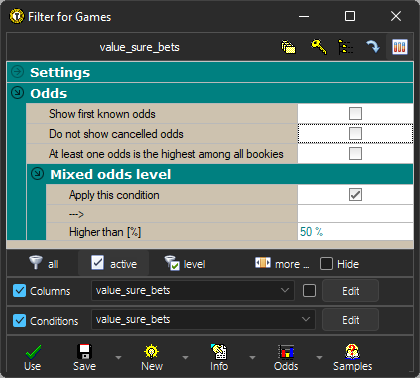The Mixed Odds Level section is also activated to display the current mixed odds level - if it were higher than 100%, then it would be a surebet. With this filter, we can search for both valuebet and surebet at the same time.

Valuebet based on the amount of odds can be calculated using the average odds of bookmakers in Trefík. Each user can choose their choice of bookmakers, which will correspond to a different valuebets search in comparison between users.

Average odds are first converted to a odds level of 100%. This means that they are increased by calculation in such a way that the mutual ratio of the odds for individual tips is preserved and that the bookmaker's margin is suppressed at the same time. We then compare the obtained increased odds with the real listed odds and look for higher odds. The entire calculation can be done in the Filter / Columns section.

In the following image, the item "*level" is marked for display first. This is the value of the Mixed Odds Level. If it is greater than 100, then it is a surebet.
The following is the calculation for the valuebet. It is divided into several steps. First, it is necessary to calculate the odds level for the average odds. To do this, we need to get the sum of the reciprocals (= 1/x) for the odds on individual picks. By default, this sum is greater than zero, and its reciprocal corresponds to the mixed odds level.

In the Columns window, we will create 3 new items that will contain the inverted values of individual average odds. The items in the picture are AVG1_X, AVG0_X and AVG2_X. Their values are defined as the reciprocals (function 1/x) of individual average odds (listed in Column 1).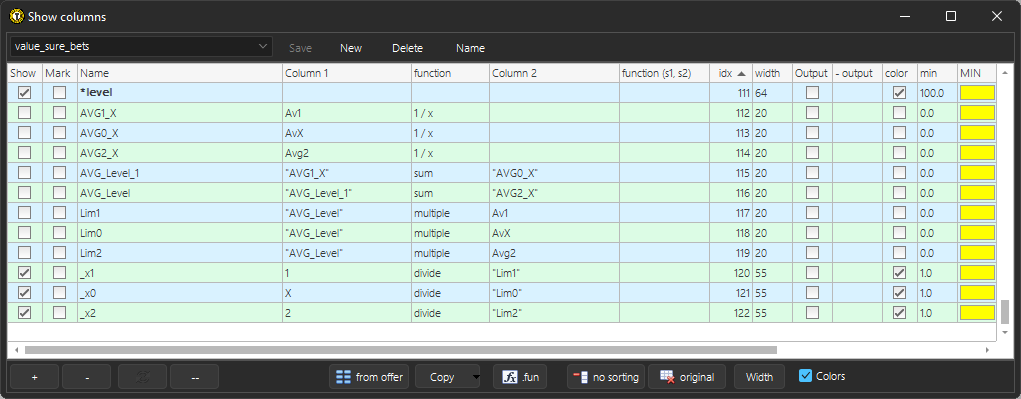Next, we need to add three new columns. Since only two values can be added together in the Filter / Columns window, we need to create one auxiliary value for this. This is labeled "AVG_Level_1" and is defined as the sum of AVG1_X and AVG0_X.
Another new AVG_Level value then sums the previous auxiliary item and the AVG2_X column. So we have the sum of the reciprocals.

And the situation is such that the sum of the inverted values just obtained indicates the coefficient by which the actual quoted odds must be multiplied in order to "disrupt" the bookmaker's margin, i.e. so that the odds level is 100%. So we create 3 more auxiliary values, each containing a multiple of the sum of the inverses obtained above and the respective average odds. This gives us Lim1, Lim0 and Lim2 odds, which are the calculated average odds when the bookmaker's margin is zero.

In the last step, we then compare the improved average odds obtained above and the actual quoted odds: we compare using a proportion so that values greater than 1 will mean that the actual quoted odds are higher than the improved average. And the calculated value will show the proportion to the improved average - which is the sought value for the valuebet.

Summary of the above:

• if the value in the *level column is higher than 100, it is a surebet,
• if the value in one of the columns x1, x0 or X2 is greater than 1, it is a valuebet.

We can use the Filter / Conditions section to display only matches that have a surebet or valuebet, and in the case of a valuebet, limit the odds on the given tip to less than 4.00 (he probably won't want to use higher odds for this bet ). We will therefore create the Filter / Conditions window as follows: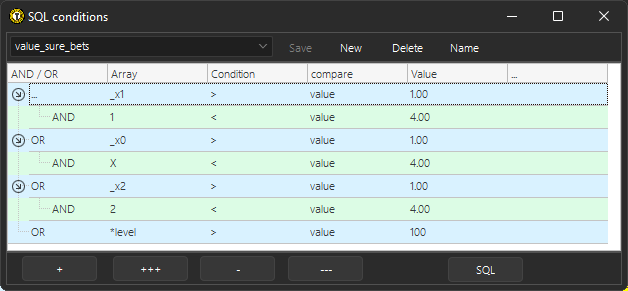.. at least one of the conditions must be met: column *level is greater than 100 OR column x1 must be greater than 1 and at the same time odds on tip 1 less than 4 OR column x0 must be greater than 1 and at the same time odds on tip 0 less than 4 OR the x2 column must be greater than 1 and at the same time the odds on tip 2 must be less than 4.

In the Offer window, the situation can then be as shown in the picture: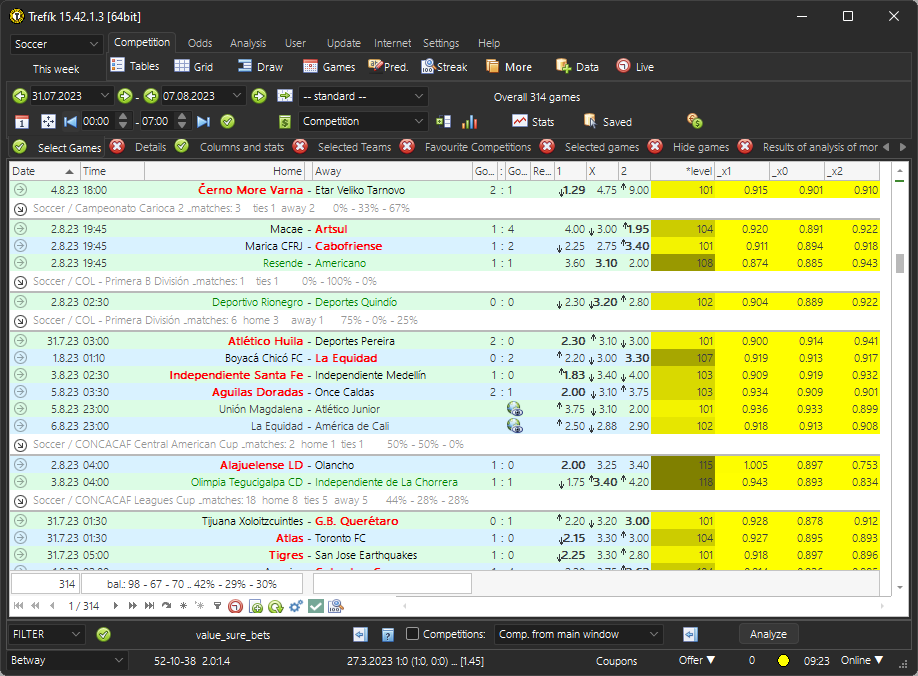.. the selected bookie at the image is Betway. If changing the bookie then the results might be different.

The above also works for other types of bets. Just change the bet type at the top of the Offer window. The next picture shows the calculation of value and surebets for the odds for the 1st half: